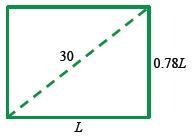### Home > PC > Chapter 10 > Lesson 10.2.2 > Problem10-92

10-92.

Find the dimensions of a rectangle whose width is $78\%$ of its length and has a diagonal of length $30$ cm?

Draw and label a diagram.Write and solve an equation using the Pythagorean Theorem.